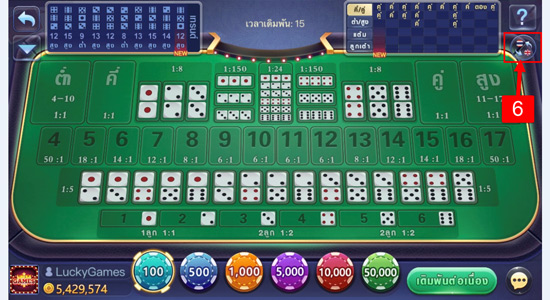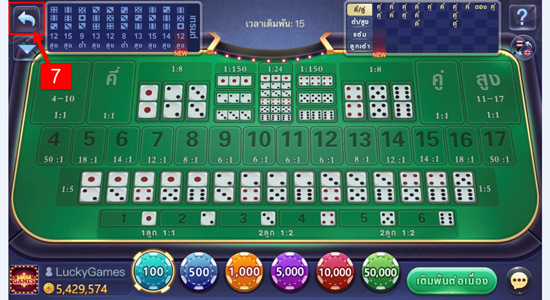# Welocome to Take Me - Live StreamSic-Bo is available now.

How To Play “Sic-Bo”
Go to TakeMe application --> Choose the game category --> Tap on "Play" Sic-Bo game.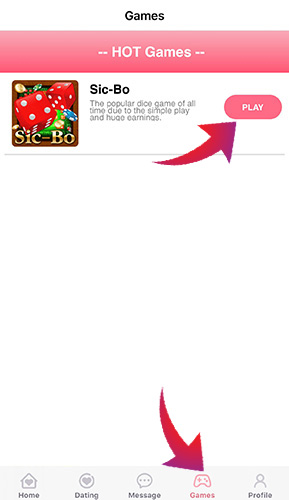How to play
1.Choose the amount of chips you want to bet.
There are 6 prices in total; 100 , 500 , 1,000 , 5,000 , 10,000 , 50,000.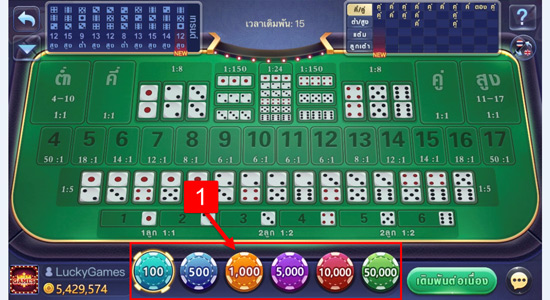2.There are 8 types of the betting in “Sic-Bo” game and the prize payout rates are different as follows.
2.1 The type of betting: High/Low.
“Low” means that the sum dice points are from 3-10 points.
“High” means that the sum dice points are from 11-17 points.
The prize payout rates of both slots are 1:1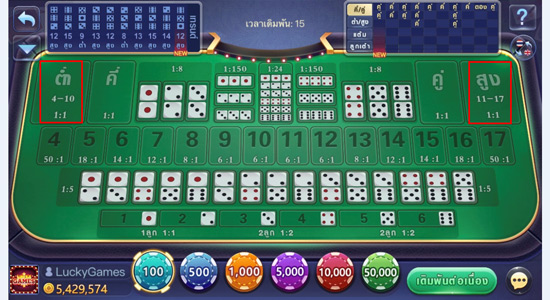2.2 The type of betting: Even/Odd.
“Even” means that the sum dice points are from 4,6,8,10,12,14,16.
“Odd” means that the sum dice points are from 5,7,9,11,13,15.
The prize payout rates of both slots are 1:1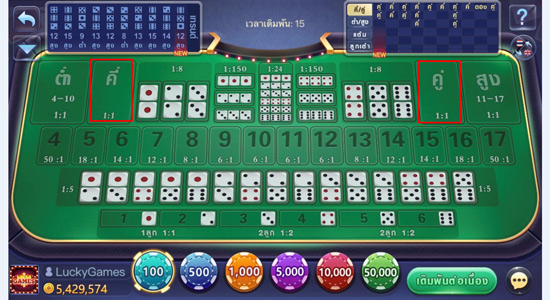2.3 The type of betting:
Predicting the total sum of dice points.
Predict 4 points หรือ 17 points: 50 times.
Predict 5 points หรือ 16 points: 18 times.
Predict 6 points หรือ 15 points: 14 times.
Predict 7 points หรือ 14 points: 12 times.
Predict 8 points หรือ 13 points: 8 times.
Predict 9 points, 10 points, 11 points, 12 points: 6 times.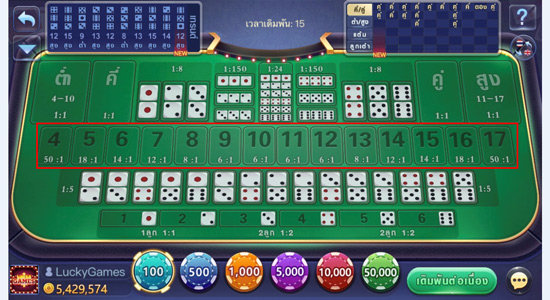2.4 The type of betting:
Predicting the numbers on two dices.
The prize payout rates of this slot are 1:5.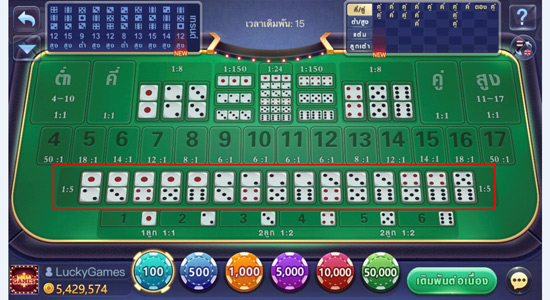2.5 The type of betting: Predicting numbers on the dices.
The prize payout rates of both slots are 1:1 , 1:2 , 1:3.
It depends on the amount of the dices
that players have predicted the dice numbers.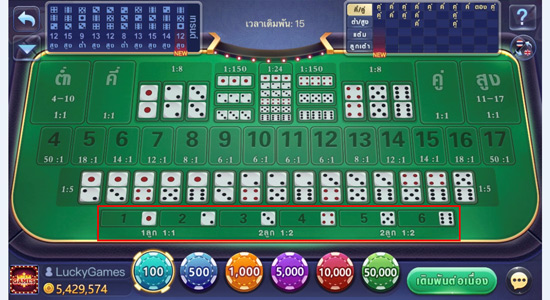2.6 The type of betting:
Predicting the same numbers on the dices
without specifying numbers.
The prize payout rates of this slot are 1:24.2.7 The type of betting:
Predicting the double numbers on the dices.
The prize payout rates of this slot are 1:8.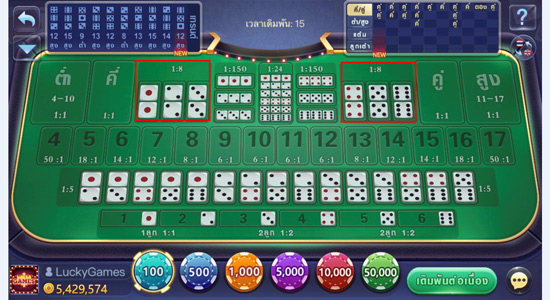2.8 The type of betting: Predicting the same numbers on the dices
with specifying numbers. The prize payout rates of this slot are 1:150.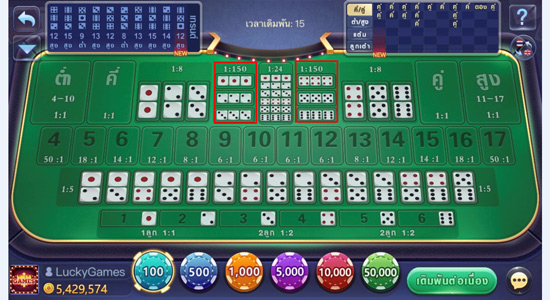3.For Continue betting, this slot means
that players will bet on the same amount
from the previous round that the players have bet on.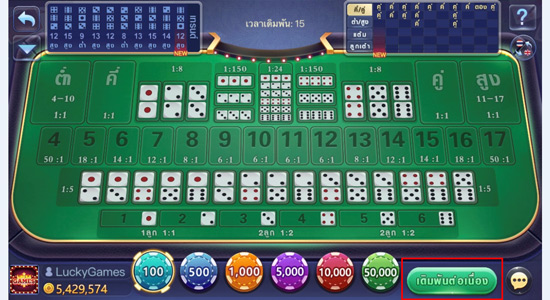4. Game Conditions.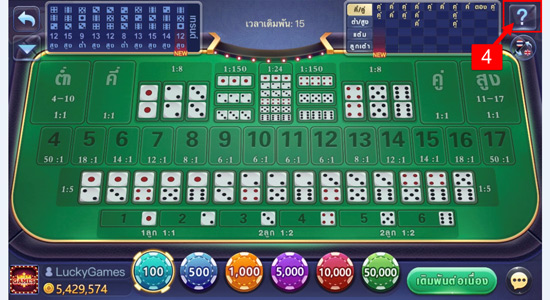5.”More” button.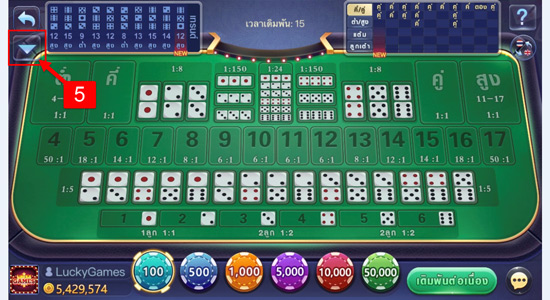5.1 “Quit Game” button.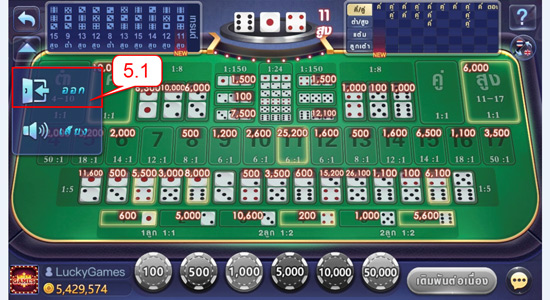5.2 “On/Off Sound” button.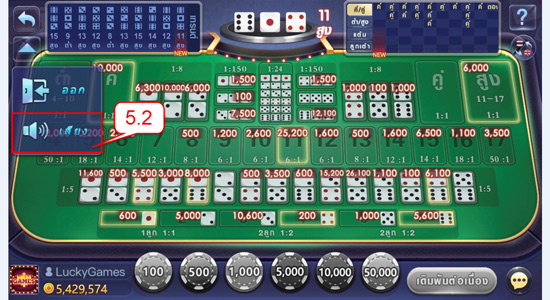6. “Language Setting” button.